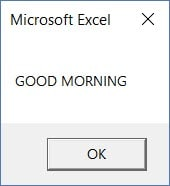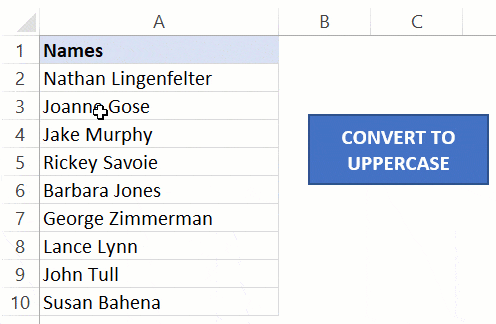VBA UCASE Function – Convert Text to Upper Case in Excel - EBOOK VBA EXCELEVBA.info ( Ebook VBA Excel ) Welcome EVBA.info - Nothing Is Unable ... About Excel Tricks, Learning VBA Programming, Dedicated Software, Free Courses, Living Skills ...

# VBA UCASE Function – Convert Text to Upper Case in Excel

In Excel worksheet, the UPPER function converts all the lowercase characters of a text string into uppercase.
There is a similar function in that also does the same – the UCase function.
The VBA UCase function takes a string as the input and converts all the lower case characters into upper case.

## Syntax of the VBA UCASE Function

Below is the syntax of the VBA UCase function
`UCase(String)`
‘String’ is the text in which you want to convert all the lowercase to uppercase.
You can use a text string, a range reference that contains the text string, or a variable that has the text string.
Let’s have a look at a couple of example of using the UCase function in Excel VBA.

## VBA UCase Examples

The below code would convert the specified text into uppercase and then display a message box with this text.
```Sub UCaseExample1()
MsgBox UCase("Good Morning")
End Sub```Below is another example VBA code, where I have used a variable (‘Var’) to hold the text string. The UCase function is then used to convert it the lower case characters into uppercase.
```Sub UCaseExample2()
Dim Var As String
Var = "Good Morning"
MsgBox UCase(Var)
End Sub```
Another example below shows how to take the string from a cell (A1) and show the uppercase text of it in a message box.
```Sub UCaseExample3()
MsgBox UCase(Range("A1"))
End Sub```
While all these above examples work, you’re unlikely to use this function to simply convert or show the uppercase string.
Below is a more practical example of the UCase function in Excel VBA.
The below code would go through all the cells in the selected range and convert all the text strings into upper case.
```Sub UCaseExample4()
Dim rng As Range
Set rng = Selection
For Each Cell In rng
Cell.Value = UCase(Cell)
Next Cell
End Sub```Here are a few important things to know about the VBA UCase function:
1. It affects only the lowercase characters of the text string. Any character other than the lowercase text characters is left unchanged. This means that numbers, special characters, and punctuations remain unchanged.
2. If you use a null character (or a reference to an empty cell), it will return a null character.
#evba #etipfree #kingexcel
📤You download App EVBA.info installed directly on the latest phone here : https://www.evba.info/p/app-evbainfo-setting-for-your-phone.html?m=1